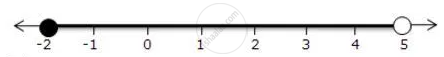Share

Represent the Following Inequalities on Real Number Lines -2 <= X < 5 - ICSE Class 10 - Mathematics

ConceptLinear Inequations in One Unknown

Question

Represent the following inequalities on real number lines

-2 <= x  < 5

Solution

-2 <= x  < 5

Solution on number line isIs there an error in this question or solution?

APPEARS IN

Solution Represent the Following Inequalities on Real Number Lines -2 <= X < 5 Concept: Linear Inequations in One Unknown.
S